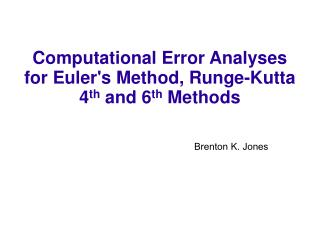DownloadDownload PresentationComputational Error Analyses for Euler's Method, Runge-Kutta 4 th and 6 th Methods

Computational Error Analyses for Euler's Method, Runge-Kutta 4 th and 6 th Methods

Télécharger la présentationComputational Error Analyses for Euler's Method, Runge-Kutta 4 th and 6 th Methods

- - - - - - - - - - - - - - - - - - - - - - - - - - - E N D - - - - - - - - - - - - - - - - - - - - - - - - - - -
Presentation Transcript

1. Computational Error Analyses for Euler's Method, Runge-Kutta 4th and 6th Methods

2. Euler's Method • Uses the first derivative to obtain slope of tangent a tangent line • Then uses the slope to approximate the value of the solution

3. Error for Euler’s method

4. Runge-Kutta Method 1 • k1 is the slope at the beginning of the interval; • k2 is the slope at the midpoint of the interval, using slope k1 to determine the value of y at the point tn + h / 2 using Euler's method; • k3 is again the slope at the midpoint, but now using the slope k2 to determine the y-value; • k4 is the slope at the end of the interval, with its y-value determined using k3.

5. Runge-Kutta Method 2 Uses trial step at midpoint of an interval to cancel out lower order error terms Estimate is determined by weighted averages of slope at midpoints Used to give numerical solutions to differential equations (approximations) Generally very accurate

6. The Solution of Harmonic Oscillator Differential Equation: y''+y=0 Initial conditions: y(0)=1, y'(0)=0 Exact Solution: y=cos t

7. Errors from the theoretical solutions with Euler’s, Runge- Kutta 4th, and Runge- Kutta 6th order.

8. Error Comparisons Euler’s vs Runge-Kutta 4th order Runge-Kutta 4th vs Runge-Kutta 6th order

9. Step sizes .005, .05, .2

10. The other example 1 Differential Equation: y''-2y'-3y=0 Initial conditions: y(0)=1, y'(0)=2 Exact Solution: 0.25 exp[-t]+0.75exp[3t]

11. Errors from the theoretical solutions with Euler’s, Runge- Kutta 4th, and Runge- Kutta 6th order.

12. Interesting Results (For this exponential solution, the 4th and 6th have similar properties in terms of the error.)

13. The other example 2 Differential Equation: u''+16u'+192u=0 Initial conditions: u(0)=0.5, u'(0)=0 Exact Solution:

14. Step size .005 Errors from the theoretical solutions with Euler’s, Runge- Kutta 4th, and Runge- Kutta 6th order.

15. The other example 3 Differential Equation: y*y''-(y')^2=1 Initial conditions: y(0)=1, y'(0)=0 Exact Solution: y=cosh(t)

16. Step size .005 Errors from the theoretical solutions with Euler’s, Runge- Kutta 4th, and Runge- Kutta 6th order.

17. Conclusions • Euler's Method (the 1st order) is the least accurate. • Runge-Kutta methods are generally very accurate. • Higher orders provide greater accuracy. • The step size greatly effects results. • Unless the solution converges to a stable solution, larger t causes larger error.